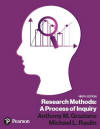﻿ Other Descriptive StatisticsGraziano & Raulin
Research Methods (9th edition)

## Other Descriptive Statistics

The most commonly used descriptive statistics for distribution of scores are the mean and the variance (or standard deviation), but there are other descriptive statistics that are available and are often computed by data analysis programs. We will discuss two of them in this section: Skewness and Kurtosis.

Skewness and kurtosis both describe an aspect of the shape of a distribution, much as the mean and the variance describe the shape of a distribution. But these indices of the shape of the distribution are used far less frequently than the mean and variance.

Skewness was discussed in the section on graphing data with histograms and frequency polygons. There is an index of the degree and direction of  skewness  that many statistical programs produce as part of their entire descriptive statistics package. When the skewness is near zero, the distribution is close to symmetric. A negative number indicates a negative skew, and a positive number indicates a positive skew.

Kurtosis indicates the degree of flatness of the distribution. The larger the number, the flatter the distribution.

Statisticians refer to a concept called moments of a distribution. The mean is based on the first moment of the distribution, and the variance is based on the second moment of the distribution. The skewness and kurtosis are based on the third and fourth moments of the distribution. These concepts make more sense to people who do theoretical work in statistics. But for the purpose of this course, the only points you need to remember is the definition of these terms in case your run across them in your reading.

If you want to compute them, most statistical analysis packages allow you to include these statistics in the descriptive statistics package.